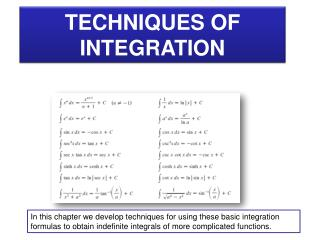DownloadDownload PresentationTECHNIQUES OF INTEGRATION

# TECHNIQUES OF INTEGRATION

Download Presentation## TECHNIQUES OF INTEGRATION

- - - - - - - - - - - - - - - - - - - - - - - - - - - E N D - - - - - - - - - - - - - - - - - - - - - - - - - - -
##### Presentation Transcript

1. TECHNIQUES OF INTEGRATION In this chapter we develop techniques for using these basic integration formulas to obtain indefinite integrals of more complicated functions.

2. INTEGRATION BY PARTS formula for integration by parts. Example Example Find Find

3. INTEGRATION BY PARTS formula for integration by parts. REMARK1: aim in using integration by parts is to obtain a simpler integral than the one we started with. REMARK2: How to choose u and dv to obtain simpler integral Example Find

4. INTEGRATION BY PARTS Example REMARK2: in some integral, we may need to apply integration by parts many times. Find

5. INTEGRATION BY PARTS Example REMARK2: in some integral, we may need to apply integration by parts many times. Find Example Find Example Find

6. INTEGRATION BY PARTS formula for integration by parts. Example Find REMARK3: sometimes a repeated application of integration by parts leads back to an integral similar to our original one. If so, this expression can be combined with original integral.

7. INTEGRATION BY PARTS Exam2 Term082 Exam2 Term102

8. Exam2 Term092

9. INTEGRATION BY PARTS Observe: Reduction Formula REMARK3: sometimes The reduction formula is useful because by using it repeatedly we could eventually express our integral.

10. INTEGRATION BY PARTS Reduction Formula Example Example

11. INTEGRATION BY PARTS Reduction Formula Example Example Reduction Formula

12. Reduction Formula

13. INTEGRATION BY PARTS Most of the time ln(x) is easier by parts Reduction Formula Integration by Parts Repeated Applications Term 0 (diff) tabular integration Back to original